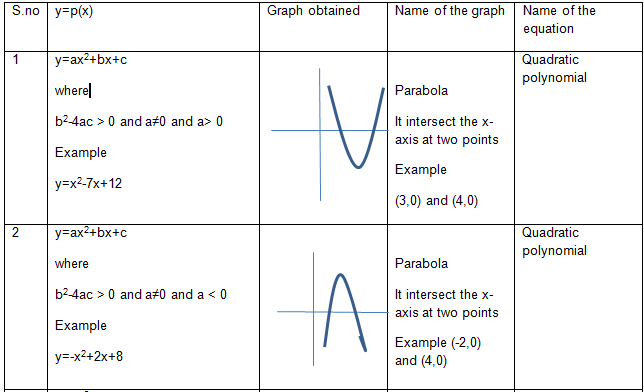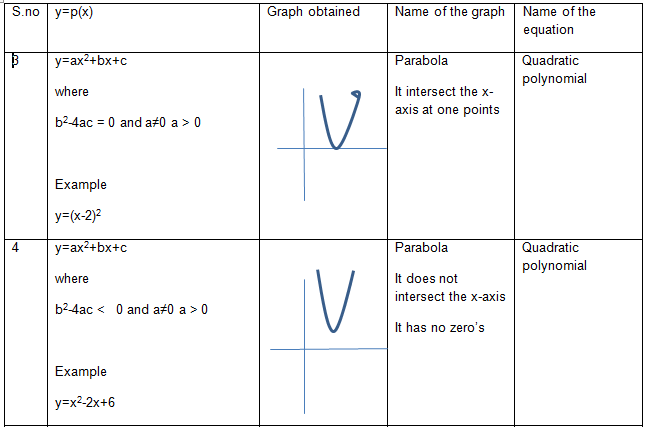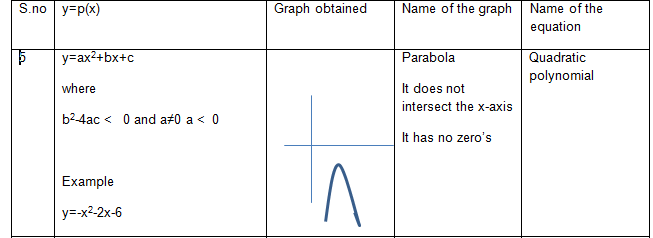P(x) = ax2 +bx+c   where a≠0

It is a polynomial of degree 2
Examples:

$P(x) =3x^2-11x-2$
$P(x)=x^2 -x -11$
$P(x)=x^2 + x -897$

Lets us assume y= p(x) where p(x) is the polynomial of quadratic type Now we can plot the equation y=p(x) on the Cartesian plane by taking various values of x and y obtained by putting the values. The plot or graph obtained can be of any shapes The zero's of the polynomial are the points where the graph meet x axis in the Cartesian plane. If the graph does not meet x axis ,then the polynomial does not have any zero's. Let us take some useful polynomial and shapes obtained on the Cartesian planeWhen we equate Quadratic Polynomial with zero, it is called Quadratic equation
$ax^2 +bx+c =0$ where a ≠0
Examples:

$6x^2-x-2=0$
$x^2 -x -20=0$
$x^2 + x -300 = 0$

It is used in many field and it has many application in Mathematics
For example
John has a area of a rectangular plot is 528 m2.The length of the plot (in meters) is one more than twice its breadth. We need to find the length and breadth of the plot
For solution , we can assume breadth is x ,then lenght would be (2x+1)
Now $528=x(2x+1)$
or $2x^2+x -528 =0$
Which is a quadratic equation and the required representation of the problem mathematically
Solution or root of the Quadratic equation
A real number $\alpha$ is called the root or solution of the quadratic equation if
$a \alpha^2 +b \alpha +c=0$

Some other points to remember
• The root of the quadratic equation is the zeroes of the polynomial p(x).
• We know from chapter two that a polynomial of degree can have max two zeroes. So a quadratic equation can have maximum two roots
• A quadratic  equation has no real roots if b2- 4ac < 0
• A graphing quadratic  equation is same as graphing quadratic polynomial as explained above

Reference Books for class 10

Given below are the links of some of the reference books for class 10 math.

You can use above books for extra knowledge and practicing different questions.

Note to our visitors :-

Thanks for visiting our website. From feedback of our visitors we came to know that sometimes you are not able to see the answers given under "Answers" tab below questions. This might happen sometimes as we use javascript there. So you can view answers where they are available by reloding the page and letting it reload properly by waiting few more seconds before clicking the button.
We really do hope that this resolve the issue. If you still hare facing problems then feel free to contact us using feedback button or contact us directly by sending is an email at [email protected]
We are aware that our users want answers to all the questions in the website. Since ours is more or less a one man army we are working towards providing answers to questions available at our website.

Practice Question

Question 1 What is $1 - \sqrt {3}$ ?
A) Non terminating repeating
B) Non terminating non repeating
C) Terminating
D) None of the above
Question 2 The volume of the largest right circular cone that can be cut out from a cube of edge 4.2 cm is?
A) 19.4 cm3
B) 12 cm3
C) 78.6 cm3
D) 58.2 cm3
Question 3 The sum of the first three terms of an AP is 33. If the product of the first and the third term exceeds the second term by 29, the AP is ?
A) 2 ,21,11
B) 1,10,19
C) -1 ,8,17
D) 2 ,11,20